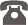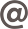FILTER

# Calculation

Information necessary for calculation

 Type Clearance Volumem3/mt Clearance Volumem2/mt Max. Concrete Heightmm D1 0,076 0,127 150 D2 0,106 0,127 150 D3 0,134 0,224 150 D4 0,160 0,267 150

Example: Concrete volume used per unit m2 is calculated as follows.

Assume that D3 moulds will be used for the floor.  Depth of D3 is 325 mm.   Assume that the upright concrete is 150 mm. Total depth would be 475 mm.

Total full volume per m2 = 1.0 x 1.0 x 0.475 = 0475 m3
Clearance volume per m2 = 0.224 m2
Concrete volume per m2 = 0.251 m2

+90 212 671 0 681